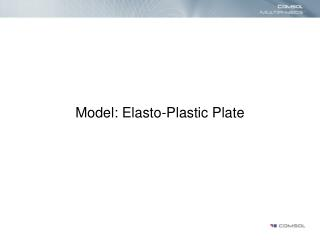Download PresentationModel: Elasto-Plastic Plate

# Model: Elasto-Plastic Plate - PowerPoint PPT PresentationDownload Presentation## Model: Elasto-Plastic Plate

- - - - - - - - - - - - - - - - - - - - - - - - - - - E N D - - - - - - - - - - - - - - - - - - - - - - - - - - -
##### Presentation Transcript

1. Model: Elasto-Plastic Plate

2. Introduction • This model treats the elastic-plastic material behavour of a plate with a centered hole. • The development of the plastic regions with increasing load are studied as well as residul stress and deformations when the plate is totally unloaded.

3. Problem Definition • The plate is thin and loaded in the plane, that is, plane stress conditions can be assumed. • Due to the double symmetry, only one quarter of the plate needs to be analyzed.

4. Problem Definition, constraints and loads. • The displacements in the normal directions are constrained at the two symmetry cuts • The right edge is subjected to a load which increases from zero to a maximum value and then released again. • The maximum load value is selected so that the mean stress over the section through the hole is 10% above the yield stress.

5. Problem Definition, material • The von Mises stress is used as yield criterion. • The model uses a isotropic hardening rule for the description of the plastic history dependent yield criterion. • Elastic properties: E=70000 N/mm2 and n=0.2 • Yield stress: sy= 243 N/mm • Tangent modulus Et= 2171 N/mm2

6. Problem Definition, material plastic s sy Elastic e

7. Problem Definition, hardening rules s sB sy Et E 2sy e Kinematic sB Isotropic

8. Results • The plastic regions (red) at peak load level where the material has exceeded the yield stress. • For a material without strain hardening, the structure would have collapsed before reaching the peak load level.

9. Results • The left figure shows the deformations and von Mises stress levels at peak load. • The right figure shows the residual deformations and the residual von Mises stress when the model is totally unloaded.